Math & Science Home | Proficiency Tests | Mathematical Thinking in Physics | Aeronauts 2000

 CONTENTS Introduction Fermi's Piano Tuner Problem How Old is Old? If the Terrestrial Poles were to Melt... Sunlight Exerts Pressure Falling Eastward What if an Asteroid Hit the Earth Using a Jeep to Estimate the Energy in Gasoline How do Police Radars really work? How "Fast" is the Speed of Light? How Long is a Light Year? How Big is a Trillion? "Seeing" the Earth, Moon, and Sun to Scale Of Stars and Drops of Water If I Were to Build a Model of the Cosmos... A Number Trick Designing a High Altitude Balloon Pressure in the Vicinity of a Lunar Astronaut Space Suit due to Outgassing of Coolant Water Calendar Calculations Telling Time by the Stars - Sidereal Time Fields, an Heuristic Approach The Irrationality ofThe Irrationality ofThe Number (i)i Estimating the Temperature of a Flat Plate in Low Earth Orbit Proving that (p)1/n is Irrational when p is a Prime and n>1 The Transcendentality ofIdeal Gases under Constant Volume, Constant Pressure, Constant Temperature and Adiabatic Conditions Maxwell's Equations: The Vector and Scalar Potentials A Possible Scalar Term Describing Energy Density in the Gravitational Field A Proposed Relativistic, Thermodynamic Four-Vector Motivational Argument for the Expression-eix=cosx+isinx Another Motivational Argument for the Expression-eix=cosx+isinx Calculating the Energy from Sunlight over a 12 hour period Calculating the Energy from Sunlight over actual full day Perfect Numbers-A Case Study Gravitation Inside a Uniform Hollow Sphere Further note on Gravitation Inside a Uniform Hollow Sphere Pythagorean Triples Black Holes and Point Set Topology Additional Notes on Black Holes and Point Set Topology Field Equations and Equations of Motion (General Relativity) The observer in modern physics A Note on the Centrifugal and Coriolis Accelerations as Pseudo Accelerations - PDF File On Expansion of the Universe - PDF File

## Field Equations & Equations of Motion (General Relativity)

Velocity is a vector (tensor) or vector (tensor) field. In familiar notation, the velocity v is represented by

v = viei

where vi represent the components of the velocity, and ei represent basis (unit) vectors in the selected coordinate system. (As usual in tensor notation, summation is assumed over all repeated indices unless otherwise specified.)

Acceleration is the first time-derivative of velocity, and involves derivatives of both the vi and the ei :

a = dv/dt = (dvi/dt)ei + vi(dei/dt) .

The second term may be further expanded as

vi(dei/dt) = vi(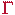ijk dxj/dt ek )

whereijk are the appropriate Christoffel symbols. Substituting, the expression for acceleration becomes

a = (dvi/dt + vssij dxj/dt )ei

(with suitable change of indices on vssij).

If, in a Euclidean space, the components of velocity, vi , are referred to an inertial (non-accelerated) Cartesian (geodesic) coordinate system, then thejik all vanish (i.e.,jik = 0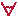values of i, j, & k) and the expression for acceleration has the form

a = (dvi/dt)ei .

If a non-Cartesian inertial coordinate system is used, say a polar or a spherical coordinate system, then thejik do not all necessarily vanish, and the expression for acceleration may involve non-zero values of some of the vssijdxj/dt ei.

[eg.: In the case of an inertial polar coordinate system, the non-zero values of vssijdxj/dt ei simply reflect the fact that the base unit vectors,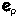and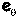depend for their direction on their location in the space. Specifically, with i and j being unit vectors in the Cartesian coordinate system, the familiar transformations are:= i sin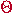+ j cos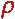, and= -i cos+ j sin,where, for a moving object,=(t) and=(t) .]

If the coordinate system to which the vi are referred is non-inertial (i.e., accelerating: say it is rotating or linearly accelerating (or both)), then thejik do not all vanish, and the expression for acceleration again involves non-zero values of the terms vssijdxj/dt ei . In this case, these non-zero values are associated with the so-called inertial accelerations, i.e., "g's", and the Coriolis and centrifugal accelerations. These accelerations are independent of any applied forces, and are due only to the accelerated motion of the coordinate system.

In a non-inertial system, the total force, ma, is the vector sum of

1. The applied force(s), m dvi/dt ei , and

2. The inertial force(s) m vssijdxj/dt ei.

Even if the applied force is zero, we still have the inertial acceleration(s):

a = vssijdxj/dt ei .

These accelerations have the characteristic that if several different test masses are sequentially placed at a point in the system, they will all experience the same inertial acceleration (i.e., the inertial force on the various test masses will be proportional to the masses only, with the acceleration being a constant). Gravitational acceleration exhibits identical behavior in this regard; i.e., in classical mechanics, the gravitational force on a body is proportional to its mass only, the acceleration being a constant at every point in the field. This observation leads to the identity of gravitational and inertial mass, noted by Newton, and used as a motivation toward General Relativity by Einstein. Let me now present a heuristic approach to the equations of General Relativity.

One method of setting up the equations of motion for bodies in classical circular orbits is to set the gravitational force equal to the centrifugal force in a coordinate system which is revolving with the body:

mg = - (mv2/r)u.

(where u is a unit vector). This expression is equivalent to setting the total force on the orbiting body equal to zero, and results in the usual equations of motion for the orbiting body:

f = mg + (mv2/r)u = 0 .

These equations may be solved if a field law is given for the gravitational field g. In classical mechanics, this law is

g = - (Gm/r2) u .

(where m is the field-generating mass).

The same reasoning may be applied to the tensor equations developed above. We first set the total force equal to zero everywhere in the gravitational field so that

dvi/dt = - vssijdxj/dt .

Using the relationship vi = dxi/dt , substituting, and rearranging terms, we then obtain

0 = d2xi/dt2 +sij(dxs/dt) (dxj/dt) ei .

This expression is the differential equation for a straight line in Euclidean space, or a geodesic in a non-Euclidean space. If the classical requirement that physical space be Euclidean is relaxed, and non-Euclidean spaces are introduced, the motion of bodies in the gravitational field may be described by this equation (equation of motion) without recourse to any gravitational 'force'; i.e., the law of motion becomes: The paths followed by bodies in a gravitational field are geodesics in a [suitable] non-Euclidean space [space-time]. The problem becomes one of properly selecting the values of thesij (components of the gravitational field). As before, this problem may be solved by specifying a field law. Einstein chose the expression

Rij - (1/2)gijR = Tij

where Rij (=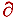h(ihj ) -j(hhi ) +hhlilj -ilhjhl , withm =/xm defined for notational convenience) is the contracted Riemann-Christoffel curvature tensor (Riss j , a.k.a. the Ricci tensor), R is the associated scalar gi jRi j , gi j is the fundamental tensor, and Ti j is the stress-energy tensor. (The expression on the l.h.s. has a vanishing divergence, satisfying the conservation of mass-energy in the gravitational field). When these equations are used with the equations of motion18

0 = d2xi/dt2 +sij (dxs/dt) (dxj/dt) .

the orbits of bodies and beams of light are accurately described.

To a first order of approximation, for speeds that are small compared with the speed of light, and mass densities which are comparable to those observed in our solar system, General Relativity gives results in agreement with the equations of Newton. When the field equations Ti j = 0 (Schwartzchild19) are used (specifying zero mass-energy density in the space surrounding the sun or any star - a good approximation for our solar system), the "classical tests" of the General Theory result: i.e.,

1. The rotation of Mercury's perihelion at a rate of 42.9 arc-sec per century (not predictable by classical mechanics unless an intra-Mercurial planet is assumed [Vulcan]);
2. The 1.75 arc-sec deflection of starlight grazing the sun's surface (measured by Eddington; originally predicted to be only half as big by classical mechanics in conjunction with Special Relativity); and
3. The red shift of starlight traveling outward in the gravitational field of a star (in agreement with classical mechanics in conjunction with Special Relativity).

Finally, for cases of very high velocities (approaching the speed of light) and/or very large mass-energy densities, the predictions of General Relativity significantly diverge from those of Newton, but are confirmable by astronomical observations.

18 In General Relativity, the differential time dt is no longer used because it is not an invariant (i.e., a scalar; it is the component of a four-vector and subject to the Lorentz transformation); rather, the invariant interval ds2= gij dxi dxj is used instead.

19 Tij=0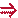Rij - (1/2)gij R=0. Multiplying the left hand side by gmi and summing yields Rjm-(1/2)jmR=0. Next, setting j = m and summing yields R - 2R = 0 or R = 0. But R = 0Rjm = 0Rij = 0 ; therefore, Tij = 0Rij = 0. The last expression is the set solved by Schwartzchild and is known as Schwartzchild's equation.